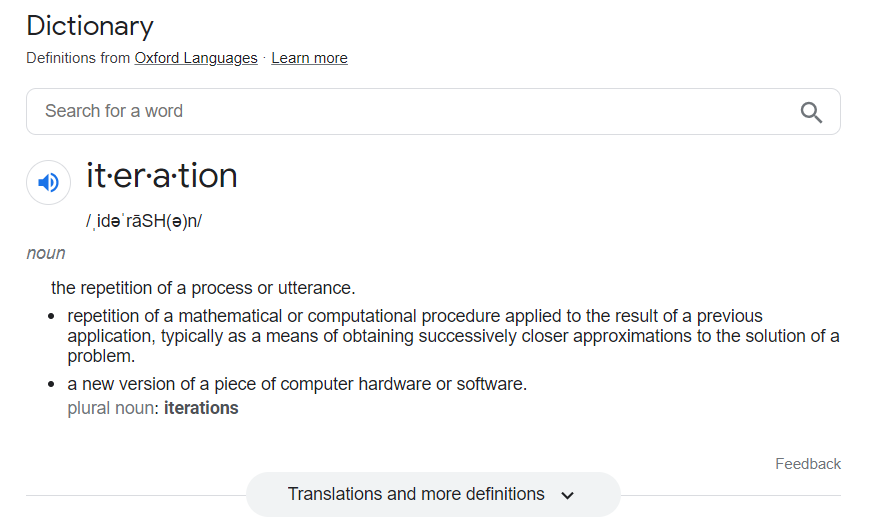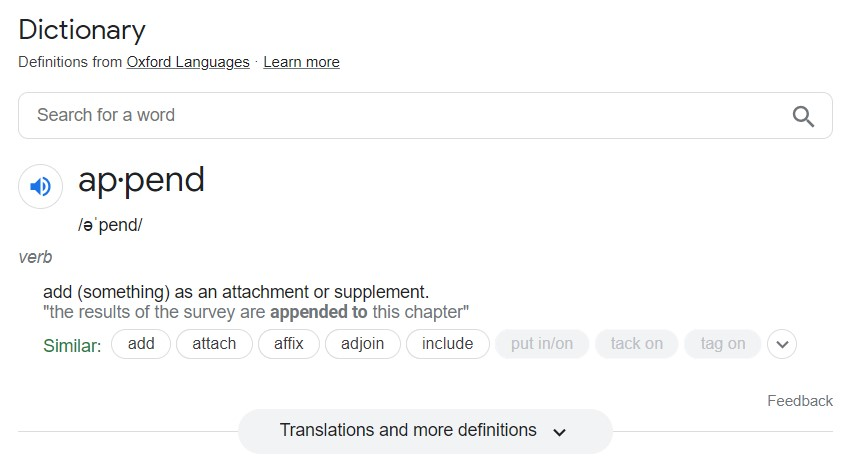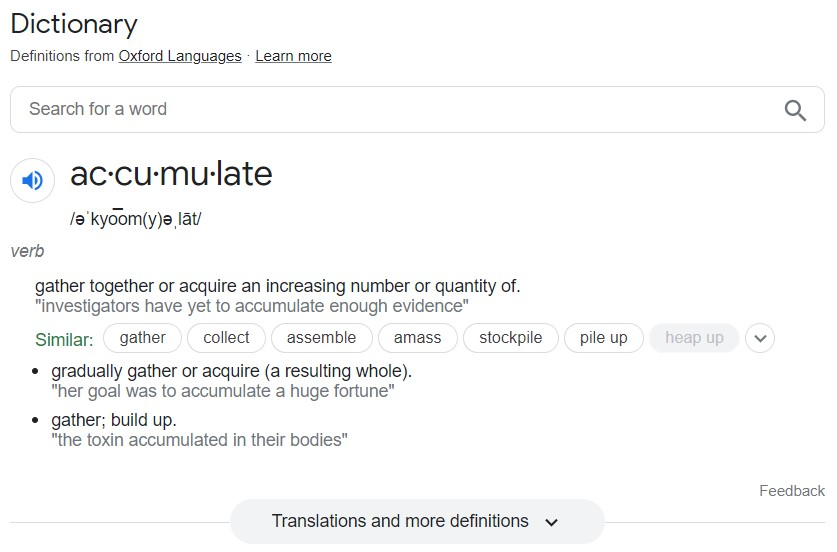# Lecture 10 – Conditional Statements and Iteration¶

## DSC 10, Spring 2023¶

### Announcements¶

• Homework 2 is due tomorrow at 11:59PM.
• Lab 3 is due on Saturday 4/29 at 11:59PM.
• Come to office hours for help! The schedule is here.
• In addition, we are offering 1-on-1 tutoring to all students in the class. 1-on-1 tutoring is meant for conceptual help, not assignment help. Look here to sign up for Week 4.
• The Midterm Project will be released tomorrow!
• You can work with a partner from any lecture section, but if you do, you must follow these project partner guidelines. In particular, you must both contribute to all parts of the project and not split up the problems.
• We will hold a mixer on Wednesday from 1:30 to 2:15PM in the Center Hall courtyard to help you find a partner if you don't have one. More details to come tomorrow.
• This Thursday from 12-1:30PM in CSE 1202, a few other DSC professors and I will be participating in a "Hot Ones"-style event hosted by DS3. Come say hi!

### Agenda¶

• Booleans.
• Conditional statements (i.e. if-statements).
• Iteration (i.e. for-loops).

Note:

• We've finished introducing new DataFrame manipulation techniques.
• Today we'll cover some foundational programming tools, which will be very relevant as we start to cover more ideas in statistics (next week).
• The last lecture that will be in scope for the Midterm Exam is Lecture 12 (this Friday).

## Booleans¶

### Recap: Booleans¶

• bool is a data type in Python, just like int, float, and str.
• It stands for "Boolean", named after George Boole, an early mathematician.
• There are only two possible Boolean values: True or False.
• Yes or no.
• On or off.
• 1 or 0.
• Comparisons result in Boolean values.

### Boolean operators; not¶

There are three operators that allow us to perform arithmetic with Booleans – not, and, and or.

not flips True ↔️ False.

### The and operator¶

The and operator is placed between two bools. It is True if both are True; otherwise, it's False.

### The or operator¶

The or operator is placed between two bools. It is True if at least one is True; otherwise, it's False.

### Order of operations¶

• By default, the order of operations is not, and, or. See the precedence of all operators in Python here.
• As usual, use (parentheses) to make expressions more clear.

### Booleans can be tricky!¶

For instance, not (a and b) is different than not a and not b! If you're curious, read more about De Morgan's Laws.

### Note: & and | vs. and and or¶

• Use the & and | operators between two Series. Arithmetic will be done element-wise (separately for each row).
• This is relevant when writing DataFrame queries, e.g. df[(df.get('capstone') == 'finished') & (df.get('units') >= 180)].
• Use the and and or operators between two individual Booleans.
• e.g. capstone == 'finished' and units >= 180.

### Concept Check ✅ – Answer at cc.dsc10.com¶

Suppose we define a = True and b = True. What does the following expression evaluate to?

not (((not a) and b) or ((not b) or a))


A. True

B. False

C. Could be either one

### Aside: The in operator¶

Sometimes, we'll want to check if a particular element is in a list/array, or a particular substring is in a string. The in operator can do this for us:

## Conditionals¶

### if-statements¶

• Often, we'll want to run a block of code only if a particular conditional expression is True.
• The syntax for this is as follows (don't forget the colon!):
if <condition>:
<body>

• Indentation matters!

### else¶

If you want to do something else if the specified condition is False, use the else keyword.

### elif¶

• What if we want to check more than one condition? Use elif.
• elif: if the specified condition is False, check the next condition.
• If that condition is False, check the next condition, and so on, until we see a True condition.
• After seeing a True condition, it evaluates the indented code and stops.
• If none of the conditions are True, the else body is run.

What if we use if instead of elif?

### Example: Percentage to letter grade¶

Below, complete the implementation of the function, grade_converter, which takes in a percentage grade (grade) and returns the corresponding letter grade, according to this table:

Letter Range
A [90, 100]
B [80, 90)
C [70, 80)
D [60, 70)
F [0, 60)

Your function should work on these examples:

>>> grade_converter(84)
'B'

'D'


def grade_converter(grade):
return 'A'
return 'B'
return 'C'
return 'D'
else:
return 'F'


### Activity¶

def mystery(a, b):
if (a + b > 4) and (b > 0):
return 'bear'
elif (a * b >= 4) or (b < 0):
return 'triton'
else:
return 'bruin'


Without running code:

1. What does mystery(2, 2) return?
2. Find inputs so that calling mystery will produce 'bruin'.

## Iteration¶### for-loops¶

• Loops allow us to repeat the execution of code. There are two types of loops in Python; the for-loop is one of them.
• The syntax of a for-loop is as follows:
for <element> in <sequence>:
<for body>

• Read this as: "for each element of this sequence, repeat this code."
• Lists, arrays, and strings are all examples of sequences.
• Like with if-statements, indentation matters!

### Example: Squares¶

The line print(num, 'squared is', num ** 2) is run four times:

• On the first iteration, num is 4.
• On the second iteration, num is 2.
• On the third iteration, num is 1.
• On the fourth iteration, num is 3.

This happens, even though there is no num = anywhere.

### Activity¶

Using the array colleges, write a for-loop that prints:

Revelle College
John Muir College
Thurgood Marshall College
Earl Warren College
Eleanor Roosevelt College
Sixth College
Seventh College

✅ Click here to see the solution after you've tried it yourself.
for college in colleges:
print(college + ' College')


### Ranges¶

• Recall, each element of a list/array has a numerical position.
• The position of the first element is 0, the position of the second element is 1, etc.
• We can write a for-loop that accesses each element in an array by using its position.
• np.arange will come in handy.

### Example: Goldilocks and the Three Bears¶

We don't have to use the loop variable!

### Randomization and iteration¶

• In the next few lectures, we'll learn how to simulate random events, like flipping a coin.
• Often, we will:
1. Run an experiment, e.g. "flip 10 coins."
2. Compute some statistic, e.g. "number of heads," and write it down somewhere.
3. Repeat steps 1 and 2 many, many times using a for-loop.### np.append¶

• This function takes two inputs:
• An array.
• An element to add on to the end of the array.
• It returns a new array. It does not modify the input array.
• We typically use it like this to extend an array by one element:
name_of_array = np.append(name_of_array, element_to_add)

• ⚠️ Remember to store the result!

### Example: Coin flipping¶

The function flip(n) flips n fair coins and returns the number of heads it saw. (Don't worry about how it works for now.)

Let's repeat the act of flipping 10 coins, 10000 times.

• Each time, we'll use the flip function to flip 10 coins and compute the number of heads we saw.
• We'll store these numbers in an array, heads_array.
• Every time we use our flip function to flip 10 coins, we'll add an element to the end of heads_array.

Now, heads_array contains 10000 numbers, each corresponding to the number of heads in 10 simulated coin flips.### The accumulator pattern¶

• To store our results, we'll typically use an int or an array.
• If using an int, we define an int variable (usually to 0) before the loop, then use + to add to it inside the loop.
• Think of this like using a tally.
• If using an array, we create an array (usually empty) before the loop, then use np.append to add to it inside the loop.
• Think of this like writing the results on a piece of paper.
• This pattern – of repeatedly adding to an int or an array – is called the accumulator pattern.

### for-loops in DSC 10¶

• Almost every for-loop in DSC 10 will use the accumulator pattern.
• Do not use for-loops to perform mathematical operations on every element of an array or Series.

• Instead use DataFrame manipulations and built-in array or Series methods.
• Helpful video 🎥: For Loops (and when not to use them) in DSC 10.

### Working with strings¶

String are sequences, so we can iterate over them, too!

### Example: Vowel count¶

Below, complete the implementation of the function vowel_count, which returns the number of vowels in the input string s (including repeats). Example behavior is shown below.

>>> vowel_count('king triton')
3

>>> vowel_count('i go to uc san diego')
8

def vowel_count(s):
# We need to keep track of the number of vowels seen so far. Before we start, we've seen zero vowels.
number = 0

# For each of the 5 vowels:
for vowel in 'aeiou':
# Count the number of occurrences of this vowel in s.
num_vowel = s.count(vowel)
# Add this count to the variable number.
number = number + num_vowel
# Once we've gotten through all 5 vowels, return the answer.
return number


## Summary, next time¶

### Summary¶

• if-statements allow us to run pieces of code depending on whether certain conditions are True.
• for-loops are used to repeat the execution of code for every element of a sequence.
• Lists, arrays, and strings are examples of sequences.

### Next time¶

• Probability.
• A math lesson – no code!